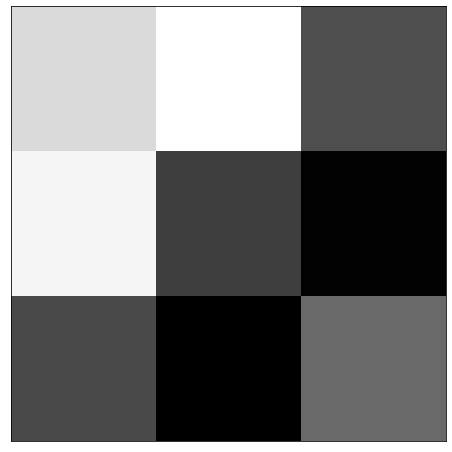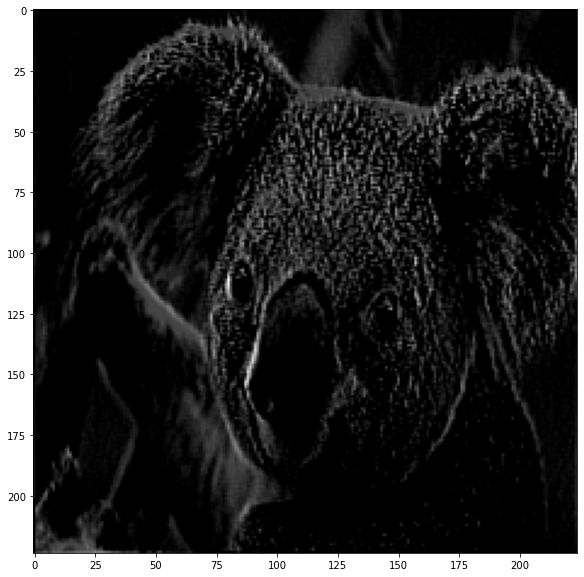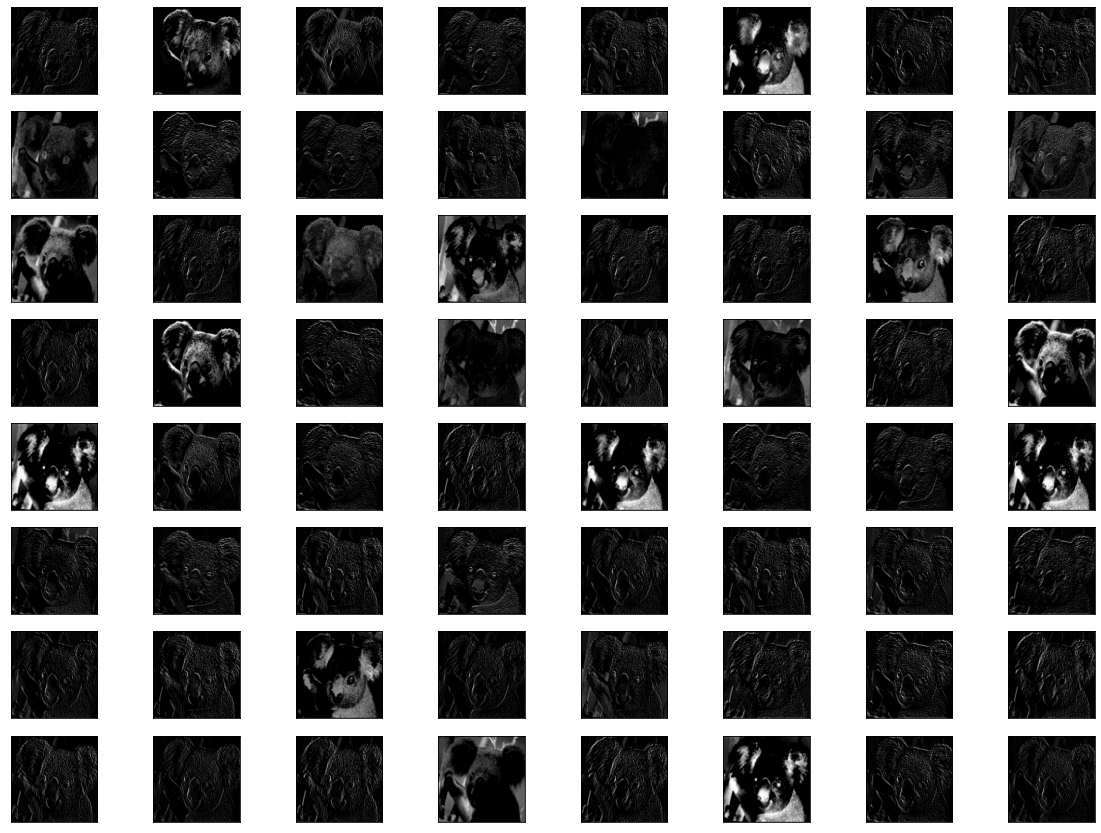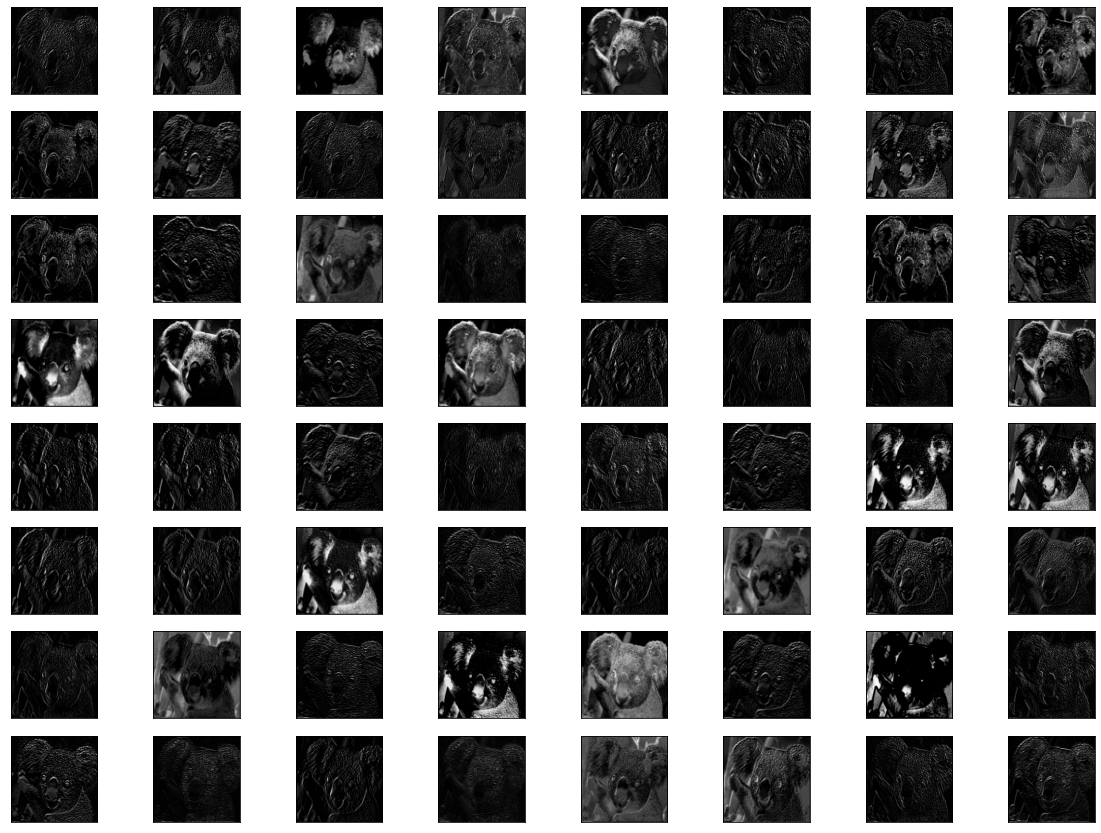### Visualizing Filters and Feature Maps in CNNs - TensorFlow Keras

In this post, we will learn how to visualize filters (weights) and feature maps in Convolutional Neural Networks (CNNs) using TensorFlow Keras. We use a pretrained model VGG16. To visualize the filters, we can directly access the filters/ weights from from the Convolutional Layers visualize the these wights using Matplotlib.

The Feature maps are the outputs from a hidden convolutional layer in the in CNNS.  To visualize these outputs in the hidden conv layers, we need to define a CNN model/ network that outputs these feature map. We will use the transfer learning for this purpose.We will visualize these feature maps using Matplotlib.

We will discuss visualizing filters and feature maps in CNNs in details in respective sections. We will cover the following topics/ table of contents. We can follow the table of contents as the steps to visualize the filters and feature maps.

This is our second post in the series of Deep Learning for Computer Vision (DL4CV).
You must have basic understanding of the Convolutional Neural Networks (CNNs), Convolutional Layer, Filters and Feature Maps.
We have implemented using the following environment/ dependencies:
• Programming Language - Python 3.8.9
• Libraries/ Packages -
• TensorFlow 2.3.0
• Matplotlib 3.4.2
• NumPy 1.20.3
• Jupyter Notebook

## Importing the Required Libraries and Modules

The first step is to import libraries and modules.
`# import vgg modelfrom tensorflow.keras.applications.vgg16 import VGG16# import Modelfrom tensorflow.keras.models import Model# imports for image preprocessingfrom tensorflow.keras.preprocessing.image import load_imgfrom tensorflow.keras.preprocessing.image import img_to_arrayimport numpy as npfrom tensorflow.keras.applications.vgg16 import preprocess_input# import marplotliobimport matplotlib.pyplot as plt`
The imports for image preprocessing are required only for visualizing the feature maps. So you can skip these imports while visualizing the filters only.

We use the VGG16 model (pretrained on ImageNet dataset) for visualizing the filters and feature maps. We have already imported the model from tensorflow.keras.applications.vgg16. We print the model summary to look different Layers are there in the model.

Load the VGG16 model as below.
`# load vgg modelmodel = VGG16()`
Output:
```A local file was found, but it seems to be incomplete or outdated because the auto file hash does not match the original value of 64373286793e3c8b2b4e3219cbf3544b so we will re-download the data.
553467904/553467096 [==============================] - 594s 1us/step```
Print the model summary as below:
`# print summary of the modelmodel.summary()`
Output:
```Model: "vgg16"
_________________________________________________________________
Layer (type)                 Output Shape              Param #
=================================================================
input_1 (InputLayer)         [(None, 224, 224, 3)]     0
_________________________________________________________________
block1_conv1 (Conv2D)        (None, 224, 224, 64)      1792
_________________________________________________________________
block1_conv2 (Conv2D)        (None, 224, 224, 64)      36928
_________________________________________________________________
block1_pool (MaxPooling2D)   (None, 112, 112, 64)      0
_________________________________________________________________
block2_conv1 (Conv2D)        (None, 112, 112, 128)     73856
_________________________________________________________________
block2_conv2 (Conv2D)        (None, 112, 112, 128)     147584
_________________________________________________________________
block2_pool (MaxPooling2D)   (None, 56, 56, 128)       0
_________________________________________________________________
block3_conv1 (Conv2D)        (None, 56, 56, 256)       295168
_________________________________________________________________
block3_conv2 (Conv2D)        (None, 56, 56, 256)       590080
_________________________________________________________________
block3_conv3 (Conv2D)        (None, 56, 56, 256)       590080
_________________________________________________________________
block3_pool (MaxPooling2D)   (None, 28, 28, 256)       0
_________________________________________________________________
block4_conv1 (Conv2D)        (None, 28, 28, 512)       1180160
_________________________________________________________________
block4_conv2 (Conv2D)        (None, 28, 28, 512)       2359808
_________________________________________________________________
block4_conv3 (Conv2D)        (None, 28, 28, 512)       2359808
_________________________________________________________________
block4_pool (MaxPooling2D)   (None, 14, 14, 512)       0
_________________________________________________________________
block5_conv1 (Conv2D)        (None, 14, 14, 512)       2359808
_________________________________________________________________
block5_conv2 (Conv2D)        (None, 14, 14, 512)       2359808
_________________________________________________________________
block5_conv3 (Conv2D)        (None, 14, 14, 512)       2359808
_________________________________________________________________
block5_pool (MaxPooling2D)   (None, 7, 7, 512)         0
_________________________________________________________________
flatten (Flatten)            (None, 25088)             0
_________________________________________________________________
fc1 (Dense)                  (None, 4096)              102764544
_________________________________________________________________
fc2 (Dense)                  (None, 4096)              16781312
_________________________________________________________________
predictions (Dense)          (None, 1000)              4097000
=================================================================
Total params: 138,357,544
Trainable params: 138,357,544
Non-trainable params: 0
_________________________________________________________________```
Notice we have five blocks of convolutional layers in the model.
We have a pre trained model, we will use the model for visualizing filters and features maps.

## How to Visualize Filters (Weights) of A CNN Layer?

Before going ahead, we must know what is filters in a CNN. A filter is a set of learnable weights which are learned using the backpropagation algorithm. You must have understood the backpropagation in Deep Learning.

Now let's move to the main part of visualizing filters.

### Access Convolution Layers

The filter is convolutional matrix of weights of a conv layer. Let's first access the convolutional layers in the model.
`for layer in model.layers:    if 'conv' not in layer.name:        continue        filter , bias = layer.get_weights()    print(layer.name , filter.shape)`
Output:
```block1_conv1 (3, 3, 3, 64)
block1_conv2 (3, 3, 64, 64)
block2_conv1 (3, 3, 64, 128)
block2_conv2 (3, 3, 128, 128)
block3_conv1 (3, 3, 128, 256)
block3_conv2 (3, 3, 256, 256)
block3_conv3 (3, 3, 256, 256)
block4_conv1 (3, 3, 256, 512)
block4_conv2 (3, 3, 512, 512)
block4_conv3 (3, 3, 512, 512)
block5_conv1 (3, 3, 512, 512)
block5_conv2 (3, 3, 512, 512)
block5_conv3 (3, 3, 512, 512)```
Look at the above output. All conv layers have 3 x 3 filters. The Fist conv layer uses such  64 filters with 3 channels whereas the second layer has 64 filters (each 3 x 3), each filter has 64 channels.

### Retrieve Filters (Weights) from A Layer

Now lets retrieve filters (weights) from a second conv layer (third hidden layer). The second conv layer has the size (3, 3, 64, 64). It has 3 x 3 filter with 64 channels.
`# retrieve weights from third layerfilters , bias = model.layers.get_weights()`
Now normalize the weights to range 0-1 so we can visualize them
`# normalize the filter values to range 0-1 so we can visualize themf_min, f_max = filters.min(), filters.max()filters = (filters - f_min) / (f_max - f_min)`
Now we have retrieved filters and normalized them. Let's move to visualize them.

### Visualize A Single Filter

Let visualize the first channel of first filer out of 64 filters.
`f = filters[:,:,:,0]fig = plt.figure(figsize=(8,8))plt.imshow(f[:,:,0],cmap='gray')plt.xticks([])plt.yticks([])#plot the filters plt.show()`
Output:Figure 1: First Channel of First Filter from VGG16- 2nd Conv Layer

You can visualize any filter from any Conv layer using the correct indexes in the above code snippets.
You can can visualize any filter with any channel with proper indexes. Suppose you want to visualize 40th channel of 17th filter, set indexes as in the code snippet.
`f = filters[:,:,:,16]fig = plt.figure(figsize=(8,8))plt.imshow(f[:,:,39],cmap='gray')plt.xticks([])plt.yticks([])#plot the filters plt.show()`

### Visualize Multiple Filters

Now move to visualize multiple filters.
Lets visualize first four filters with first three channels as below:
`n_filters, ix =4, 1fig = plt.figure(figsize=(10,10))for i in range(n_filters):    # get the filters    f = filters[:,:,:,i]    for j in range(3):        # subplot for 3 filters and 3 channels        plt.subplot(n_filters,3,ix)        plt.imshow(f[:,:,j], cmap='gray')        ix+=1#plot the filters plt.show()`
OutputFigure 2: First Four Filters with 3 Channel from VGG16

We have 64 filters and each having 64 channels in the second conv layers, we visualize the first four of 64 filters.
Try to visualize all 64 filters from the first Conv Layer (the second layer).

### Complete Program

Below is the complete program to visualize the first four of 64 filters from third hidden layer (second conv layer) of VGG16 pre trained model.
`# import vgg modelfrom tensorflow.keras.applications.vgg16 import VGG16# import marplotliobimport matplotlib.pyplot as plt# import Modelfrom tensorflow.keras.models import Model# from tensorflow.keras.preprocessing.image import load_img# from tensorflow.keras.preprocessing.image import img_to_array# import numpy as np# load vgg modelmodel = VGG16()# print summary of the model# model.summary()# Access Convutional Layersfor layer in model.layers:    if 'conv' not in layer.name:        continue        filter , bias = layer.get_weights()    #print(layer.name , filter.shape)    # retrieve weights (filters) from third hidden layerfilters , bias = model.layers.get_weights()# normalize the filter values to range 0-1 so we can visualize themf_min, f_max = filters.min(), filters.max()filters = (filters - f_min) / (f_max - f_min)# visualize multiple filtersn_filters, ix =4, 1fig = plt.figure(figsize=(10,10))for i in range(n_filters):     # get the filters    f = filters[:,:,:,i]    for j in range(3):         # subplot for 3 filters and 3 channels        plt.subplot(n_filters,3,ix)        plt.imshow(f[:,:,j], cmap='gray')        ix+=1#plot the filters plt.show()`
Now we have visualized the filters in a CNN (VGG16) using TensorFlow Keras.
Now let move on to the second part of the post- How to visualize Feature Maps.

## How to Visualize Feature Maps

We can't directly visualize the feature maps as we did for filters. To access feature maps we need define a custom model that outputs the particular feature maps. Suppose we want to visualize the feature maps in the second conv layer (third layer). For this we could redefine the model to output at second conv layer.

We will visualize the filters from second conv layer ( the third layer) in VGG16 model.

### Summarize Feature Map Size for Each Conv Layer

Lets first summarize the feature map size for each Conv layer as below:
`for i in range(len(model.layers)):    layer = model.layers[i]    if 'conv' not in layer.name:        continue    print(i , layer.name , layer.output.shape)`
Output:
```1 block1_conv1 (None, 224, 224, 64)
2 block1_conv2 (None, 224, 224, 64)
4 block2_conv1 (None, 112, 112, 128)
5 block2_conv2 (None, 112, 112, 128)
7 block3_conv1 (None, 56, 56, 256)
8 block3_conv2 (None, 56, 56, 256)
9 block3_conv3 (None, 56, 56, 256)
11 block4_conv1 (None, 28, 28, 512)
12 block4_conv2 (None, 28, 28, 512)
13 block4_conv3 (None, 28, 28, 512)
15 block5_conv1 (None, 14, 14, 512)
16 block5_conv2 (None, 14, 14, 512)
17 block5_conv3 (None, 14, 14, 512)```
Notice 17 Conv layers within five blocks. Look at the output shape of each of layers. The first Conv layer has 64 feature maps each of size 224 x 224. We will use the above information to define a new model that output the same as the output of a hidden Conv layer.

### Redefine Model to Output Right After a Hidden Layer

Let's now define a new model. The output of this model is the same as the output of first Conv layer ( overall 2nd layer in original model - Load vgg model). Input to our new model is same as the input of original model as we loaded model in step - Load vgg model.
`model = Model(inputs=model.inputs , outputs=model.layers.output)`
Now we have our new custom model. The output of this model is the same as the output first Conv layer in VGG16 model.

The outputs of any Conv Layer are the feature maps.
So the feature maps of fist Conv Layer in VGG16 model will be same the output of our above redefined model.
The next step towards the visualizing the feature maps, we need an input image and pass through the redefined model.

### Load input image and preprocess

We will use the below image as the input to our redefined model.Figure 3: Koala.jpg

We do the following in this step:
• We load the image "Koala.jpg" and reshape as the input shape of the model.
• Convert the loaded image to an array and
• Expand the dimensions so that it represents a batch of single image. The input to the redefined model is same as original model. The model takes input of batch of images.
• Prepare the image for redefined vgg model, i.e. scale the pixel values.
`# load image with required shapeimage = load_img("koala.jpg" , target_size=(224,224))# convert the image to an arrayimage = img_to_array(image)# expand dimensions so that it represents a single 'sample'image = np.expand_dims(image, axis=0)# prepare the image for vgg model ie scale pixel valuesimage = preprocess_input(image)`
Now we loaded the image preprocess suitable to input to the model.

### Get the Feature Map (Prediction from Custom Model)

Now the time to get the feature maps. It is the the output of Conv layer or the same as output of our redefined custom model.
`#calculating features_mapfeatures = model.predict(image)`

### Visualize a Single Feature Map

Let's visualize a single feature map.
`#calculating features_mapfeatures = model.predict(image)fig = plt.figure(figsize=(10,10))plt.imshow(features[0,:,:,2] , cmap='gray')    plt.show()`Figure 4: Visualization of First Feature Map (3rd Channel) From Second Convolution Layer in VGG16 Model

We visualized the third channel of the first feature map.

### Visualize Multiple Feature Maps

Use the below code snippet to visualize all 64 feature maps from the first Conv layer.
`#Visualize the Feature Mapsfig = plt.figure(figsize=(20,15))for i in range(1,features.shape+1):     plt.subplot(8,8,i)    plt.xticks([])    plt.yticks([])    plt.imshow(features[0,:,:,i-1] , cmap='gray')plt.show()`
Output:Figure 5: Feature Maps From First Convolution Layer in VGG16 Model

Similarly you have redefine the model to visualize feature maps from other Conv layer. Look at the following feature maps from the 2nd Conv layer.Figure 6: Feature Maps From Second Convolution Layer in VGG16 Model

### Complete Program To Visualize Feature Maps

Look at the below program to visualize the feature maps from the 2nd Conv Layer of VGG16.
`# import vgg modelfrom tensorflow.keras.applications.vgg16 import VGG16, preprocess_input# import Modelfrom tensorflow.keras.models import Model# imports for image preprocessingfrom tensorflow.keras.preprocessing.image import load_imgfrom tensorflow.keras.preprocessing.image import img_to_arrayimport numpy as npfrom tensorflow.keras.applications.vgg16 import preprocess_input# import marplotliobimport matplotlib.pyplot as plt#Load vgg Modelmodel = VGG16()# design a new model that is a subset of the layers in the full VGG16 model.# The new model would have the same input layer as the original model, # but the output would be the output of a given convolutional layer (here layer 3)model2 = Model(inputs=model.inputs , outputs=model.layers.output)# load input imageimage = load_img("koala.jpg" , target_size=(224,224))# convert the image to an arrayimage = img_to_array(image)# expand dimensions so that it represents a single 'sample'image = np.expand_dims(image, axis=0)# prepare the image for vgg model ie scale pixel valuesimage = preprocess_input(image)#calculating features_mapfeatures = model2.predict(image)# visualize featrure mapsfig = plt.figure(figsize=(20,15))for i in range(1,features.shape+1):    plt.subplot(8,8,i)    plt.xticks([])    plt.yticks([])    plt.imshow(features[0,:,:,i-1] , cmap='gray')plt.show()`
We completed all task listed in the table of content. Hope you like this post. This is the second post in the series of Deep Learning for Computer Vision (DL4CV). We have our first post in the series as Image Classification with CIFAR10 Dataset using TensorFlow Keras